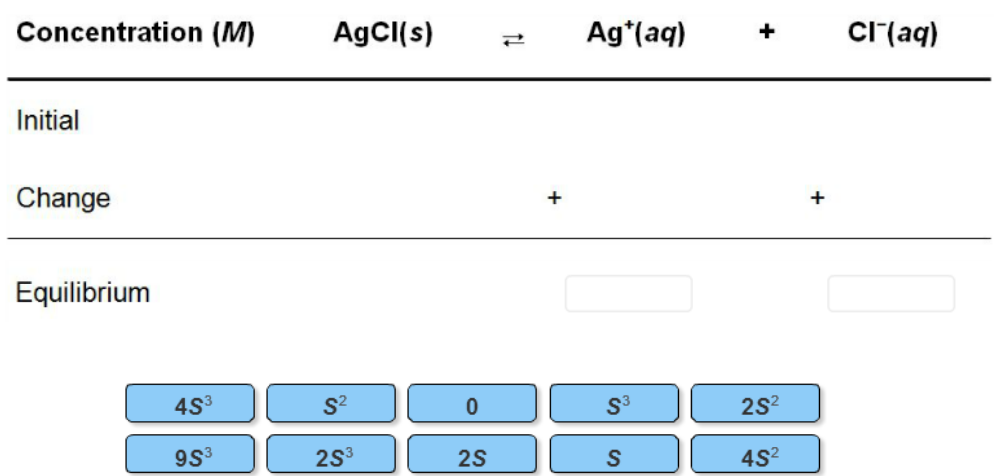# Problem: Complete the following reaction table and Ksp expression to calculate the solubility of silver chloride.

79% (34 ratings)
###### Problem Details

Complete the following reaction table and Ksp expression to calculate the solubility of silver chloride.What scientific concept do you need to know in order to solve this problem?

Our tutors have indicated that to solve this problem you will need to apply the Ksp concept. You can view video lessons to learn Ksp. Or if you need more Ksp practice, you can also practice Ksp practice problems.

What is the difficulty of this problem?

Our tutors rated the difficulty ofComplete the following reaction table and Ksp expression to ...as medium difficulty.

How long does this problem take to solve?

Our expert Chemistry tutor, Dasha took 2 minutes and 7 seconds to solve this problem. You can follow their steps in the video explanation above.

What professor is this problem relevant for?

Based on our data, we think this problem is relevant for Professor Liu's class at JMU.